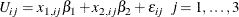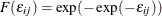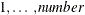# The MDC Procedure

### Conditional Logit: Estimation and Prediction

The MDC procedure is similar in use to the other regression model procedures in the SAS System. However, the MDC procedure requires identification and choice variables. For example, consider a random utility functionwhere the cumulative distribution function of the stochastic component is a Type I extreme value,. You can estimate this conditional logit model with the following statements:

   proc mdc;
model decision = x1 x2 / type=clogit
choice=(mode 1 2 3);
id pid;
run;


Note that the MDC procedure, unlike other regression procedures, does not include the intercept term automatically. The dependent variable decision takes the value 1 when a specific alternative is chosen; otherwise, it takes the value 0. Each individual is allowed to choose one and only one of the possible alternatives. In other words, the variable decision takes the value 1 one time only for each individual. If each individual has three elements (1, 2, and 3) in the choice set, the NCHOICE=3 option can be specified instead of CHOICE=(mode 1 2 3).

Consider the following trinomial data from Daganzo (1979). The original data (origdata) contain travel time (ttime1–ttime3) and choice (choice) variables. The variables ttime1–ttime3 are the travel times for three different modes of transportation, and choice indicates which one of the three modes is chosen. The choice variable must have integer values.

data origdata;
input ttime1 ttime2 ttime3 choice @@;
datalines;
16.481  16.196  23.89   2  15.123  11.373  14.182  2
19.469  8.822   20.819  2  18.847  15.649  21.28   2
12.578  10.671  18.335  2  11.513  20.582  27.838  1
10.651  15.537  17.418  1  8.359   15.675  21.05   1

... more lines ...



A new data set (newdata) is created because PROC MDC requires that each individual decision maker has one case for each alternative in his choice set. Note that the ID statement is required for all MDC models. In the following example, there are two public transportation modes, 1 and 2, and one private transportation mode, 3, and all individuals share the same choice set.

The first nine observations of the raw data set are shown in Figure 18.1.

Figure 18.1: Initial Choice Data

Obs ttime1 ttime2 ttime3 choice
1 16.481 16.196 23.890 2
2 15.123 11.373 14.182 2
3 19.469 8.822 20.819 2
4 18.847 15.649 21.280 2
5 12.578 10.671 18.335 2
6 11.513 20.582 27.838 1
7 10.651 15.537 17.418 1
8 8.359 15.675 21.050 1
9 11.679 12.668 23.104 1

The following statements transform the data according to MDC procedure requirements:

data newdata(keep=pid decision mode ttime);
set origdata;
array tvec{3} ttime1 - ttime3;
retain pid 0;
pid + 1;
do i = 1 to 3;
mode = i;
ttime = tvec{i};
decision = ( choice = i );
output;
end;
run;


The first nine observations of the transformed data set are shown in Figure 18.2.

Figure 18.2: Transformed Modal Choice Data

Obs pid mode ttime decision
1 1 1 16.481 0
2 1 2 16.196 1
3 1 3 23.890 0
4 2 1 15.123 0
5 2 2 11.373 1
6 2 3 14.182 0
7 3 1 19.469 0
8 3 2 8.822 1
9 3 3 20.819 0

The decision variable, decision, must have one nonzero value for each decision maker that corresponds to the actual choice. When the RANK option is specified, the decision variable must contain rank data. For more details, see the section MODEL Statement. The following SAS statements estimate the conditional logit model by using maximum likelihood:

proc mdc data=newdata;
model decision = ttime /
type=clogit
nchoice=3
optmethod=qn
covest=hess;
id pid;
run;


The MDC procedure enables different individuals to have different choice sets. When all individuals have the same choice set, the NCHOICE= option can be used instead of the CHOICE= option. However, the NCHOICE= option is not allowed when a nested logit model is estimated. When the NCHOICE=number option is specified, the choices are generated as. For more flexible alternatives (for example, 1, 3, 6, 8), you need to use the CHOICE= option. The choice variable must have integer values.

The OPTMETHOD=QN option specifies the quasi-Newton optimization technique. The covariance matrix of the parameter estimates is obtained from the Hessian matrix because COVEST=HESS is specified. You can also specify COVEST=OP or COVEST=QML. See the section MODEL Statement for more details.

The MDC procedure produces a summary of model estimation displayed in Figure 18.3. Since there are multiple observations for each individual, the "Number of Cases" (150)—that is, the total number of choices faced by all individuals—is larger than the number of individuals, "Number of Observations" (50).

Figure 18.3: Estimation Summary Table

The MDC Procedure

Conditional Logit Estimates

Model Fit Summary
Dependent Variable decision
Number of Observations 50
Number of Cases 150
Log Likelihood -33.32132
Log Likelihood Null (LogL(0)) -54.93061
Maximum Absolute Gradient 2.97024E-6
Number of Iterations 6
Optimization Method Dual Quasi-Newton
AIC 68.64265
Schwarz Criterion 70.55467

Figure 18.4 shows the frequency distribution of the three choice alternatives. In this example, mode 2 is most frequently chosen.

Figure 18.4: Choice Frequency

Discrete Response Profile
Index CHOICE Frequency Percent
0 1 14 28.00
1 2 29 58.00
2 3 7 14.00

The MDC procedure computes nine goodness-of-fit measures for the discrete choice model. Seven of them are pseudo-R-square measures based on the null hypothesis that all coefficients except for an intercept term are zero (Figure 18.5). McFadden’s likelihood ratio index (LRI) is the smallest in value. For more details, see the section Model Fit and Goodness-of-Fit Statistics.

Figure 18.5: Likelihood Ratio Test and R-Square Measures

Goodness-of-Fit Measures
Measure Value Formula
Likelihood Ratio (R) 43.219 2 * (LogL - LogL0)
Upper Bound of R (U) 109.86 - 2 * LogL0
Aldrich-Nelson 0.4636 R / (R+N)
Cragg-Uhler 1 0.5787 1 - exp(-R/N)
Cragg-Uhler 2 0.651 (1-exp(-R/N)) / (1-exp(-U/N))
Estrella 0.6666 1 - (1-R/U)^(U/N)
Adjusted Estrella 0.6442 1 - ((LogL-K)/LogL0)^(-2/N*LogL0)
McFadden's LRI 0.3934 R / U
Veall-Zimmermann 0.6746 (R * (U+N)) / (U * (R+N))

Finally, the parameter estimate is displayed in Figure 18.6.

Figure 18.6: Parameter Estimate of Conditional Logit

The MDC Procedure

Conditional Logit Estimates

Parameter Estimates
Parameter DF Estimate Standard
Error
t Value Approx
Pr > |t|
ttime 1 -0.3572 0.0776 -4.60 <.0001

The predicted choice probabilities are produced using the OUTPUT statement:

   output out=probdata pred=p;


The parameter estimates can be used to forecast the choice probability of individuals that are not in the input data set. To do so, you need to append to the input data set extra observations whose values of the dependent variable decision are missing, since these extra observations are not supposed to be used in the estimation stage. The identification variable pid must have values that are not used in the existing observations. The output data set, probdata, contains a new variable, p, in addition to input variables in the data set, extdata.

The following statements forecast the choice probability of individuals that are not in the input data set:

data extra;
input pid mode decision ttime;
datalines;
51  1  .   5.0
51  2  .  15.0
51  3  .  14.0
;

data extdata;
set newdata extra;
run;

proc mdc data=extdata;
model decision = ttime /
type=clogit
covest=hess
nchoice=3;
id pid;
output out=probdata pred=p;
run;

proc print data=probdata( where=( pid >= 49 ) );
var mode decision p ttime;
id pid;
run;


The last nine observations from the forecast data set (probdata ) are displayed in Figure 18.7. It is expected that the decision maker will choose mode "1" based on predicted probabilities for all modes.

Figure 18.7: Out-of-Sample Mode Choice Forecast

pid mode decision p ttime
49 1 0 0.46393 11.852
49 2 1 0.41753 12.147
49 3 0 0.11853 15.672
50 1 0 0.06936 15.557
50 2 1 0.92437 8.307
50 3 0 0.00627 22.286
51 1 . 0.93611 5.000
51 2 . 0.02630 15.000
51 3 . 0.03759 14.000